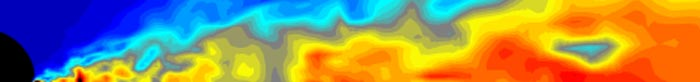Fall 2008: Special Relativity and Waves (171.201/171.207)

Overview: Special Relativity and Waves is the third course in the four-semester introductory sequence for physics majors. The course is divided into two parts. In the first three weeks we study the theory of special relativity (this is where 171.207 course ends after the first midterm and relevant homework). Then the rest of the semester is devoted to the physics of waves (for those who take the full course 171.201). The course builds upon the background in classical mechanics and electromagnetism, and precedes the full development of quantum physics.

Required texts:

• "Introduction to Special Relativity"
Author: Robert Resnick
Published: John Wiley and Sons
• "The Physics of Vibrations and Waves," 6th edition
Author: H. J. Pain
Published: John Wiley and Sons

Part I: Special Relativity

Lecture 1 (Sep. 5, 2008): Introduction: course overview, handouts:
Syllabus
Preliminary schedule
Galilean transformations, Maxwell equations, problems with relativity in EM. Michelson-Morley experiment. Postulates of Special Relativity.
Chapter 1 of Resnick

Lecture 2 (Sep. 8, 2008): Postulates of Special Relativity. Lorentz transformations. Length contraction, time dilation.
Chapters 2.1-2.3 of Resnick

Lecture 3 (Sep. 10, 2008): Lorentz transformations. More examples. Minkowski space-time.
Chapters 2.4-2.5, A.1-A.2 of Resnick

Lecture 4 (Sep. 12, 2008): More on Minkowski space-time. Twin paradox and "time travel."
Chapters A.3, B of Resnick

Lecture 5 (Sep. 15, 2008): Addition of Velocities. Doppler effect and Aberration.
Chapters 2.6-2.8 of Resnick

Lecture 6 (Sep. 17, 2008): Relativistic dynamics. Equivalence of mass and energy, relativistic mass.
Chapters 3.1-3.5 of Resnick

Lecture 7 (Sep. 19, 2008): Charged particle in Electric and magnetic fields. Transformation of momentum, energy, and mass between frames.
Chapters 3.5, 3.7 of Resnick

Lecture 8 (Sep. 22, 2008): More examples of relativistic dynamics. EM theory and relativity.
Chapters 3.6-3.7, 4.1-4.2 of Resnick

Lecture 9 (Sep. 24, 2008): Force transformation. EM field transformation and field of a moving charge.
Chapters 4.3-4.4 of Resnick

Lecture 10 (Sep. 26, 2008): Photon and EM effects. Equivalence principle of General Relativity.
Chapters 4, C (selected topics) of Resnick

Part II: Waves

Lecture 11 (Sep. 29, 2008): Intro to Waves. Simple harmonic oscillations. Complex numbers. Energy of a harmonic oscillator.
Chapter 1 of Pain

Lecture 12 (Oct. 1, 2008): Damped harmonic oscillations. Demos.
Chapter 2 of Pain

Lecture 13 (Oct. 3, 2008): Quality factor of damped harmonic oscillations. Superposition of waves. Demos.
Chapter 2 of Pain

Lecture 14 (Oct. 6, 2008): Electric circuits. Forced oscillations. Demos.
Chapter 3 of Pain

Lecture 15 (Oct. 8, 2008): More on forced oscillations.
Chapter 3 of Pain

Lecture 16 (Oct. 10, 2008): MIDTERM EXAM on Special Relativity

No Lecture (Oct. 13, 2008): Fall Day Break

Lecture 17 (Oct. 15, 2008): Coupled oscillations.
Chapter 4 of Pain

Lecture 18 (Oct. 17, 2008): More on coupled oscillations, forced coupled oscillations, many-body coupled oscillations.
Chapter 4 of Pain

Lecture 19 (Oct. 20, 2008): Many-body coupled oscillations and wave equation.
Chapters 4 and beginning of 5 of Pain

Lecture 20 (Oct. 22, 2008): Waves. Progressive waves. Standing waves.
Chapter 5 of Pain

Lecture 21 (Oct. 23, 2008): Impedance of a string. Transmission and reflection of waves.
Chapter 5 of Pain

Lecture 22 (Oct. 27, 2008): Matching impedance. Group and phase velocity.
Chapter 5 of Pain

Lecture 23 (Oct. 29, 2008): More on group and phase velocity. Energy in a normal mode of a standing wave. Idea of Fourier series.
Chaptera 5 and beginning of 10 of Pain

Lecture 24 (Oct. 31, 2008): Fourier series. Application to standing waves.
Chapter 10 of Pain

Lecture 25 (Nov. 3, 2008): Fourier transformations.
Chapter 10 of Pain

Lecture 26 (Nov. 5, 2008): Applications of Fourier transformations.
Chapters 10 and 5 of Pain

Lecture 27 (Nov. 7, 2008): More on Fourier transformations. The uncertainty principle. Sound waves in gases.
Chapters 10 and 5, touch on Chapters 13, Move to Chapter 6 of Pain

Lecture 28 (Nov. 10, 2008): More on sound waves.
Chapter 6 of Pain

Lecture 29 (Nov. 12, 2008): More on sound waves. Transmission lines.
Chapters 6, 7 of Pain

Lecture 30 (Nov. 14, 2008): MIDTERM EXAM on Waves

Lecture 31 (Nov. 17, 2008): More on transmission lines. Resistance in transmission lines. Waves in 2D.
Chapter 7 of Pain

Lecture 32 (Nov. 19, 2008): Waves in 2D and 3D. Waveguides. Separation of variables. Standing waves in 2D.
Chapter 9 of Pain

Lecture 32 (Nov. 21, 2008): Electromagnetic waves.
Chapter 8 of Pain

Lecture 33 (Nov. 24, 2008): Electromagnetic waves.
Chapter 8 of Pain

No Lecture (Nov. 26, 2008): Section canceled

Lecture 34 (Dec. 1, 2008): EM waves, interference, diffraction,
Chapter 8, 12 of Pain

Lecture 35 (Dec. 3, 2008): EM waves, interference, diffraction,
Chapter 12 of Pain

Lecture 36 (Dec. 5, 2008): EM waves, interference, diffraction,
Chapter 12 of Pain

No Lecture (Dec. 8, 2008): Review section (Bloomberg 278 and 172)

FINAL EXAM (Dec. 17, 2008)

Andrei Gritsan
Last update: Fri Nov 28 18:45:10 EST 2008